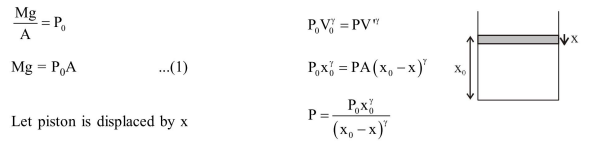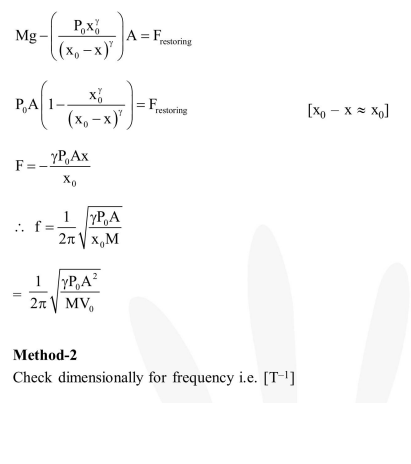# An ideal gas enclosed in a vertical cylindrical container supports a freely moving piston of mass M.

Question:

An ideal gas enclosed in a vertical cylindrical container supports a freely moving piston of mass M. The piston and the cylinder have equal cross sectional area $A$. When the piston is in equilibrium, the volume of the gas is $\mathrm{V}_{0}$ and its pressure is $\mathrm{P}_{0}$. The piston is slightly displaced from the equilibrium position and released. Assuming that the system is completely isolated from its surrounding, the piston executes a simple harmonic motion with frequency.

1. $\frac{1}{2 \pi} \frac{A \gamma P_{0}}{V_{0} M}$

2. $\frac{1}{2 \pi} \frac{V_{0} M_{0}}{A^{2} \gamma}$

3. $\frac{1}{2 \pi} \sqrt{\frac{\mathrm{A}^{2} \gamma \mathrm{P}_{0}}{\mathrm{MV}_{0}}}$

4. $\frac{1}{2 \pi} \sqrt{\frac{\mathrm{MV}_{0}}{\mathrm{A \gamma \textrm {P } _ { 0 }}}}$

Correct Option: , 2

Solution: